# mlpt

Multiscale local 1-D polynomial transform

## Syntax

``````[coefs,T,coefsPerLevel,scalingMoments] = mlpt(x,t)``````
``````[coefs,T,coefsPerLevel,scalingMoments] = mlpt(x,t,numLevel)``````
``````[coefs,T,coefsPerLevel,scalingMoments] = mlpt(x)``````
``````[coefs,T,coefsPerLevel,scalingMoments] = mlpt(___,Name,Value)``````

## Description

example

``````[coefs,T,coefsPerLevel,scalingMoments] = mlpt(x,t)``` returns the multiscale local polynomial 1-D transform (MLPT) of input signal `x` sampled at the sampling instants, `t`. If `x` or `t` contain `NaN`s, the union of the `NaN`s in `x` and `t` is removed before obtaining the `mlpt`.```

example

``````[coefs,T,coefsPerLevel,scalingMoments] = mlpt(x,t,numLevel)``` returns the transform for `numLevel` resolution levels.```

example

``````[coefs,T,coefsPerLevel,scalingMoments] = mlpt(x)``` uses uniform sampling instants for `x` as the time instants if `x` does not contain `NaN`s. If `x` contains `NaN`s, the `NaN`s are removed from `x` and the nonuniform sampling instants are obtained from the numeric elements of `x`.```

example

``````[coefs,T,coefsPerLevel,scalingMoments] = mlpt(___,Name,Value)``` specifies `mlpt` properties using one or more `Name,Value` pair arguments and any of the previous input arguments.```

## Examples

collapse all

Create a signal with nonuniform sampling and verify good reconstruction when performing the `mlpt` and `imlpt`.

Create and plot a sine wave with non-uniform sampling.

```timeVector = 0:0.01:1; sineWave = sin(2*pi*timeVector)'; samplesToErase = randi(100,100,1); sineWave(samplesToErase) = []; timeVector(samplesToErase) = []; figure(1) plot(timeVector,sineWave,'o') hold on```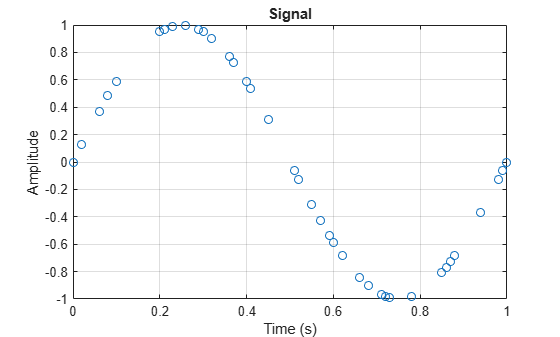Perform the multiscale local 1-D polynomial transform (`mlpt`) on the signal. Visualize the coefficients.

```[coefs,T,coefsPerLevel,scalingMoments] = mlpt(sineWave,timeVector); figure(2) stem(coefs) title('Wavelet Coefficients')```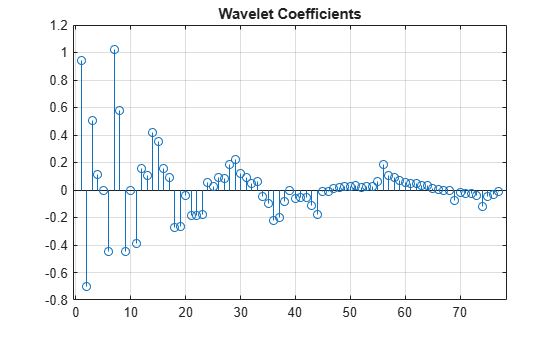Perform the inverse multiscale local 1-D polynomial transform (`imlpt`) on the coefficients. Visualize the reconstructed signal.

```y = imlpt(coefs,T,coefsPerLevel,scalingMoments); figure(1) plot(T,y,'*') legend('Original Signal','Reconstructed Signal') hold off```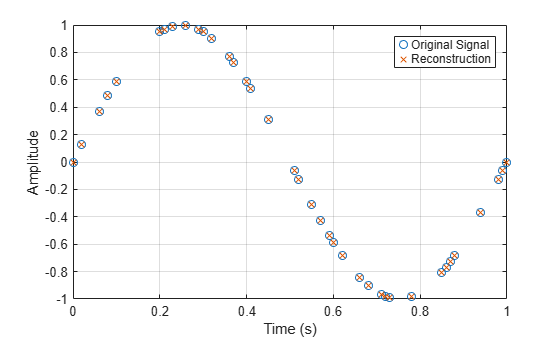Look at the total error to verify good reconstruction.

`reconstructionError = sum(abs(y-sineWave))`
```reconstructionError = 3.2547e-15 ```

Specify nondefault dual moments by using the `mlpt` function. Compare the results of analysis and synthesis using the default and nondefault dual moments.

Create an input signal and visualize it.

```T = (1:16)'; x = T.^2; plot(x) hold on```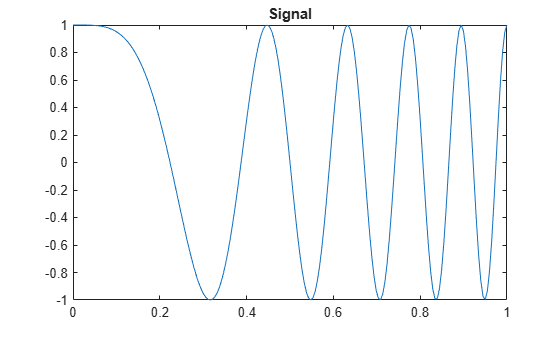Perform the forward and inverse transform for the input signal using the default and nondefault dual moments.

```[w2,t2,nj2,scalingmoments2] = mlpt(x,T); y2 = imlpt(w2,t2,nj2,scalingmoments2); [w3,t3,nj3,scalingmoments3] = mlpt(x,T,'dualmoments',3); y3 = imlpt(w3,t3,nj3,scalingmoments3,'dualmoments',3);```

Plot the reconstructed signal and verify perfect reconstruction using both the default and nondefault dual moments.

```plot(y2,'o') plot(y3,'*') legend('Original Signal', ... 'DualMoments = 3', ... 'DualMoments = 2 (Default)'); fprintf('\nMean Reconstruction Error:\n');```
```Mean Reconstruction Error: ```
`fprintf(' - Nondefault dual moments: %0.2f\n',mean(abs(y3-x)));`
``` - Nondefault dual moments: 0.00 ```
`fprintf(' - Default dual moments: %0.2f\n\n',mean(abs(y2-x)));`
``` - Default dual moments: 0.00 ```
`hold off`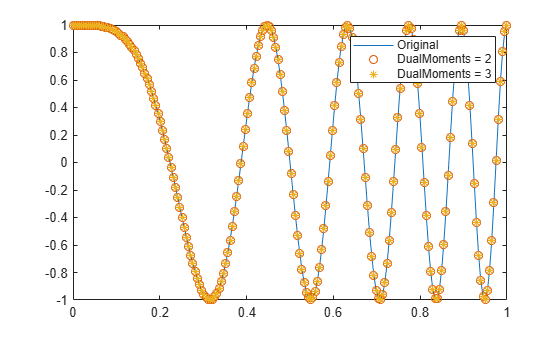Resolution levels are the number of cascaded local polynomial smoothing operations. The details at each resolution level are obtained by predicting one half the samples based on a local polynomial interpolation of the other half. The difference between the predicted and actual values are the details at each resolution level. The scaling coefficients at each coarser resolution level are smoother versions of the higher resolution scaling coefficients. Only the final-level scaling coefficients are retained.

Increasing the number of resolution levels enables you to analyze narrowband coefficients for a computational and memory cost.

Create a dual-tone input signal, `x`, that contains high and low frequencies.

```fs = 1000; t = (0:1/fs:10)'; x = sin(499*pi.*t) + sin(2*pi.*t);```

Use `mlpt` to obtain coefficients for minimum and maximum resolution levels. Print the computation time.

```tic [w1,~,nj1,m1] = mlpt(x,t,1); computationTime1 = toc; fprintf('Level one computation time: %0.2f\n',computationTime1)```
```Level one computation time: 1.57 ```
```tic [w13,~,nj13,m13] = mlpt(x,t,13); computationTime13 = toc; fprintf('Level thirteen computation time: %0.2f\n',computationTime13)```
```Level thirteen computation time: 2.14 ```

If your time instants are not known or specified, you can calculate the MLPT using default time instants.

Load a data signal corrupted with NaNs and with unknown time instants. Calculate the MLPT without specifying time instants. The resulting implied time instants is a vector of valid indices of the corrupted signal.

```load('CorruptedData.mat'); [w,t,nj,scalingMoments] = mlpt(yCorrupt);```

Calculate the inverse MLPT and visualize the results. Reinsert NaNs to visualize gaps in the signal.

```z = imlpt(w,t,nj,scalingMoments); zToPlot = NaN(numel(yCorrupt),1); zToPlot(t) = z; plot(yCorrupt,'k','LineWidth',2.5) hold on plot(zToPlot,'c','LineWidth',1) hold off legend('Original Signal','Reconstructed Signal') xlabel('Time Instants')```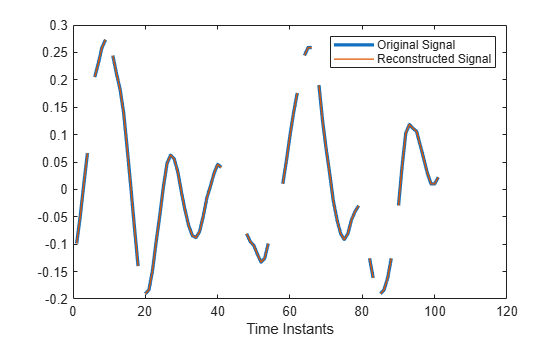## Input Arguments

collapse all

Input signal, specified as a vector or matrix.

• matrix — `x` must have at least two rows. `mlpt` operates independently on each column of `x`. The number of elements in `t` must equal the row dimension of `x`. Any `NaN`s in the columns of `x` must occur in the same rows.

• vector — `x` and `t` must have the same number of elements.

Data Types: `double`

Sampling instants corresponding to the input signal, specified as a vector, `duration` array, or `datetime` array of monotonically increasing real values. The default value depends on the length of the input signal, `x`.

Data Types: `double` | `duration` | `datetime`

Number of resolution levels, specified as a positive integer. The maximum value of `numLevel` depends on the shape of the input signal, `x`:

• matrix — `floor(log2(size(x,1)))`

• vector — `floor(log2(length(x)))`

If `numLevel` is not specified, `mlpt` uses the maximum value.

Data Types: `double`

### Name-Value Arguments

Specify optional pairs of arguments as `Name1=Value1,...,NameN=ValueN`, where `Name` is the argument name and `Value` is the corresponding value. Name-value arguments must appear after other arguments, but the order of the pairs does not matter.

Before R2021a, use commas to separate each name and value, and enclose `Name` in quotes.

Example: `'DualMoments',3` computes a transform using three dual vanishing moments.

Number of dual vanishing moments in the lifting scheme, specified as the comma-separated pair consisting of `'DualMoments'` and `2`, `3` or `4`.

Data Types: `double`

Number of primal vanishing moments in the lifting scheme, specified as the comma-separated pair consisting of `'PrimalMoments'` and `2`, `3`, or `4`.

Data Types: `double`

Prefilter before `mlpt` operation, specified as the comma-separated pair consisting of `'Prefilter'` and`'Haar'` , `'UnbalancedHaar'`, or `'None'`.

Data Types: `char` | `string`

## Output Arguments

collapse all

MLPT coefficients, returned as a vector or matrix of coefficients, depending on the level to which the transform is calculated. `coefs` contains the approximation and detail coefficients.

Data Types: `double`

Sampling instants corresponding to output, returned as a vector or `duration` array of sample times obtained from `x` and `t`. The `imlpt` function requires `T` as an input. If the input `t` is a `datetime` or `duration` array, `t` is converted to units that allow for the stable computation of the `mlpt` and `imlpt`. Then `T` is returned as a `duration` array.

Data Types: `double` | `duration`

Coefficients per resolution level, returned as a vector containing the number of coefficients at each resolution level in `coefs`. The elements of `coefsPerLevel` are organized as follows:

• `coefsPerLevel(1)` — Number of approximation coefficients at the coarsest resolution level.

• `coefsPerLevel(i)` — Number of detail coefficients at resolution level `i`, where ```i = numLevel – i + 2``` for ```i = 2, ..., numLevel + 1```.

The smaller the index `i`, the lower the resolution. The MLPT is two times redundant in the number of detail coefficients, but not in the number of approximation coefficients.

Data Types: `double`

Scaling function moments, returned as a `length(coefs)`-by-`P` matrix, where `P` is the number of primal moments specified by the `PrimalMoments` name-value pair.

Data Types: `double`

## Algorithms

Maarten Jansen developed the theoretical foundation of the multiscale local polynomial transform (MLPT) and algorithms for its efficient computation . The MLPT uses a lifting scheme, wherein a kernel function smooths fine-scale coefficients with a given bandwidth to obtain the coarser resolution coefficients. The `mlpt` function uses only local polynomial interpolation, but the technique developed by Jansen is more general and admits many other kernel types with adjustable bandwidths .

 Jansen, Maarten. “Multiscale Local Polynomial Smoothing in a Lifted Pyramid for Non-Equispaced Data.” IEEE Transactions on Signal Processing 61, no. 3 (February 2013): 545–55. https://doi.org/10.1109/TSP.2012.2225059.

 Jansen, Maarten, and Mohamed Amghar. “Multiscale Local Polynomial Decompositions Using Bandwidths as Scales.” Statistics and Computing 27, no. 5 (September 2017): 1383–99. https://doi.org/10.1007/s11222-016-9692-8.

 Jansen, Maarten, and Patrick Oonincx. Second Generation Wavelets and Applications. London ; New York: Springer, 2005.